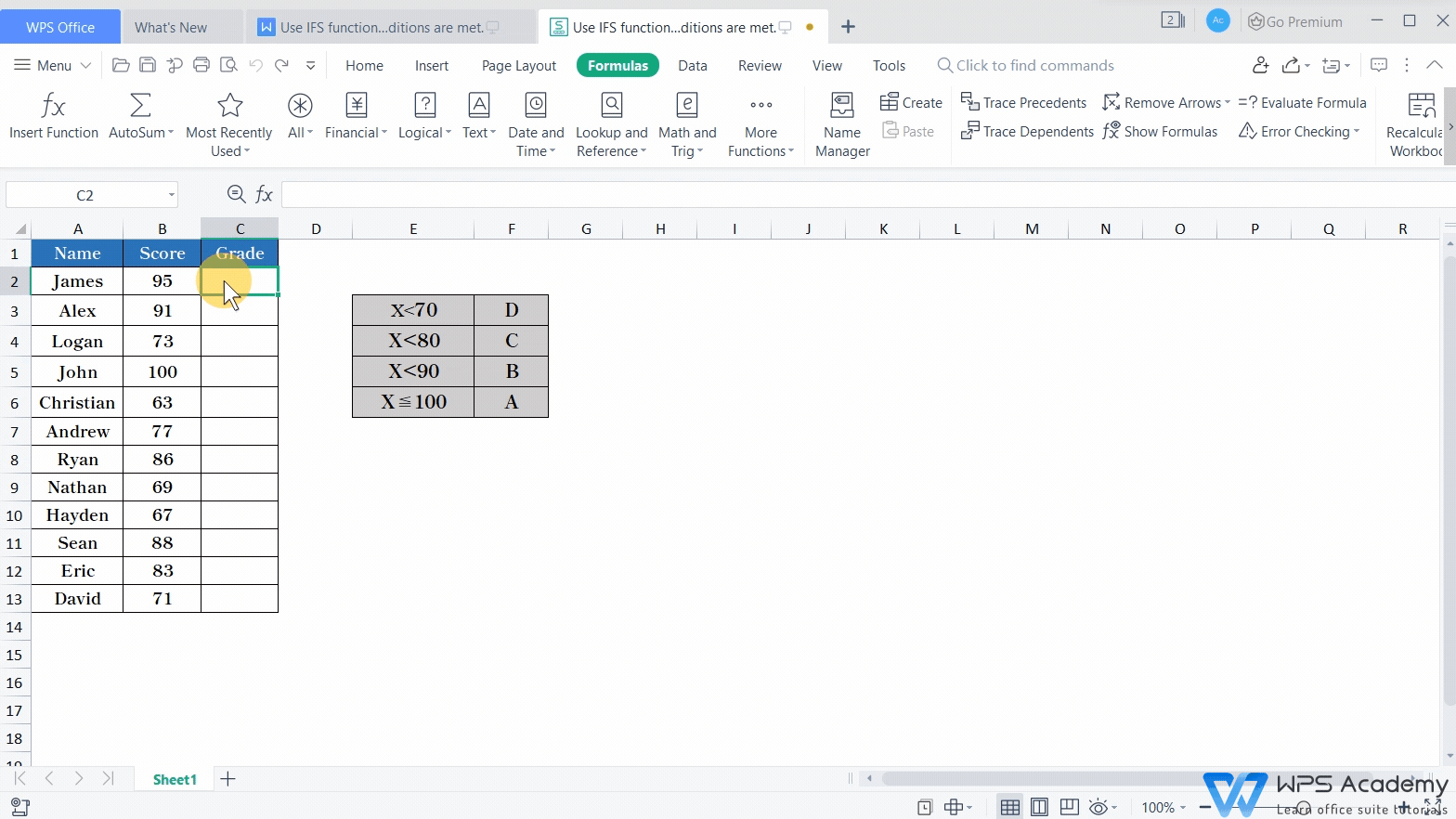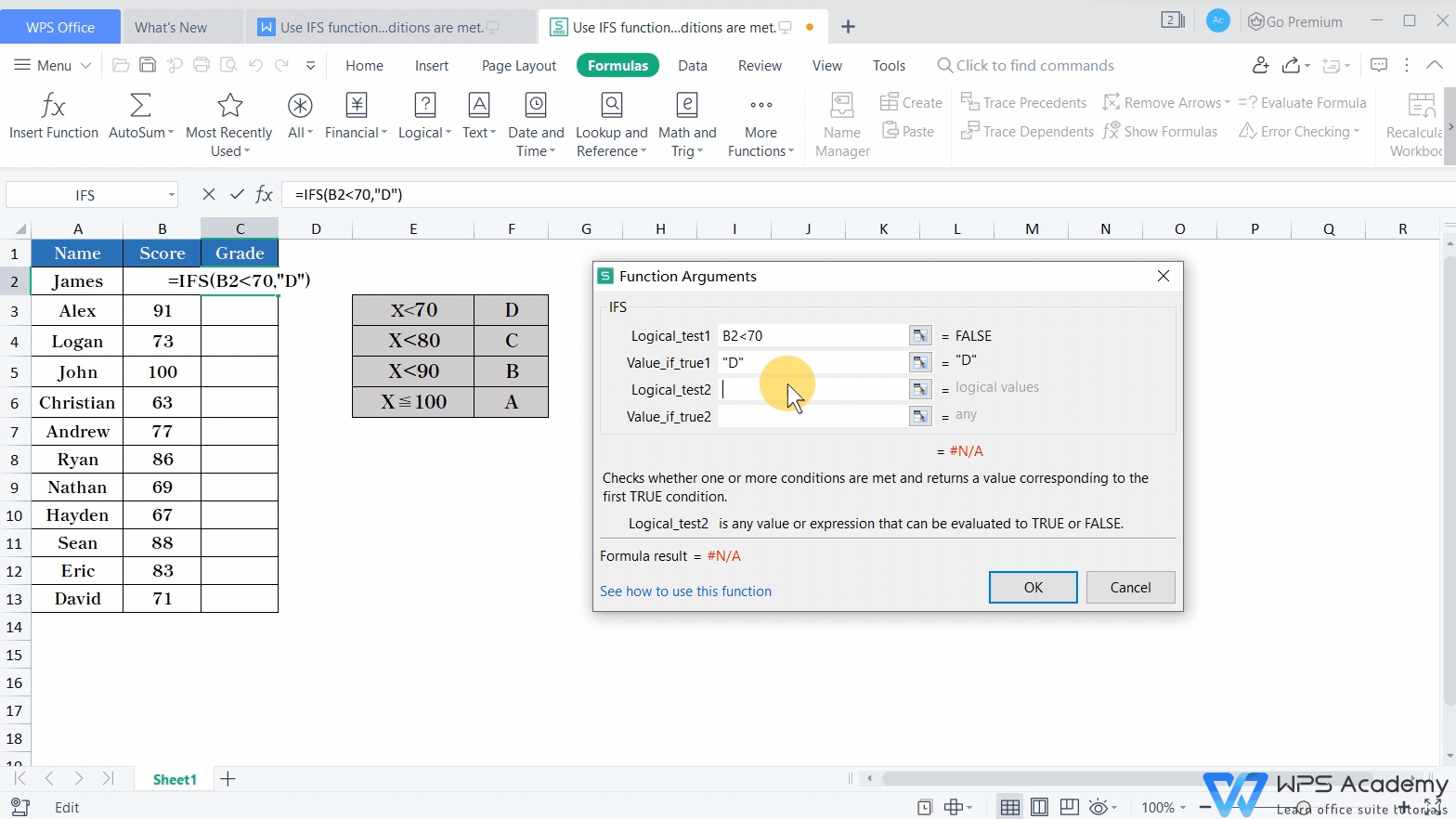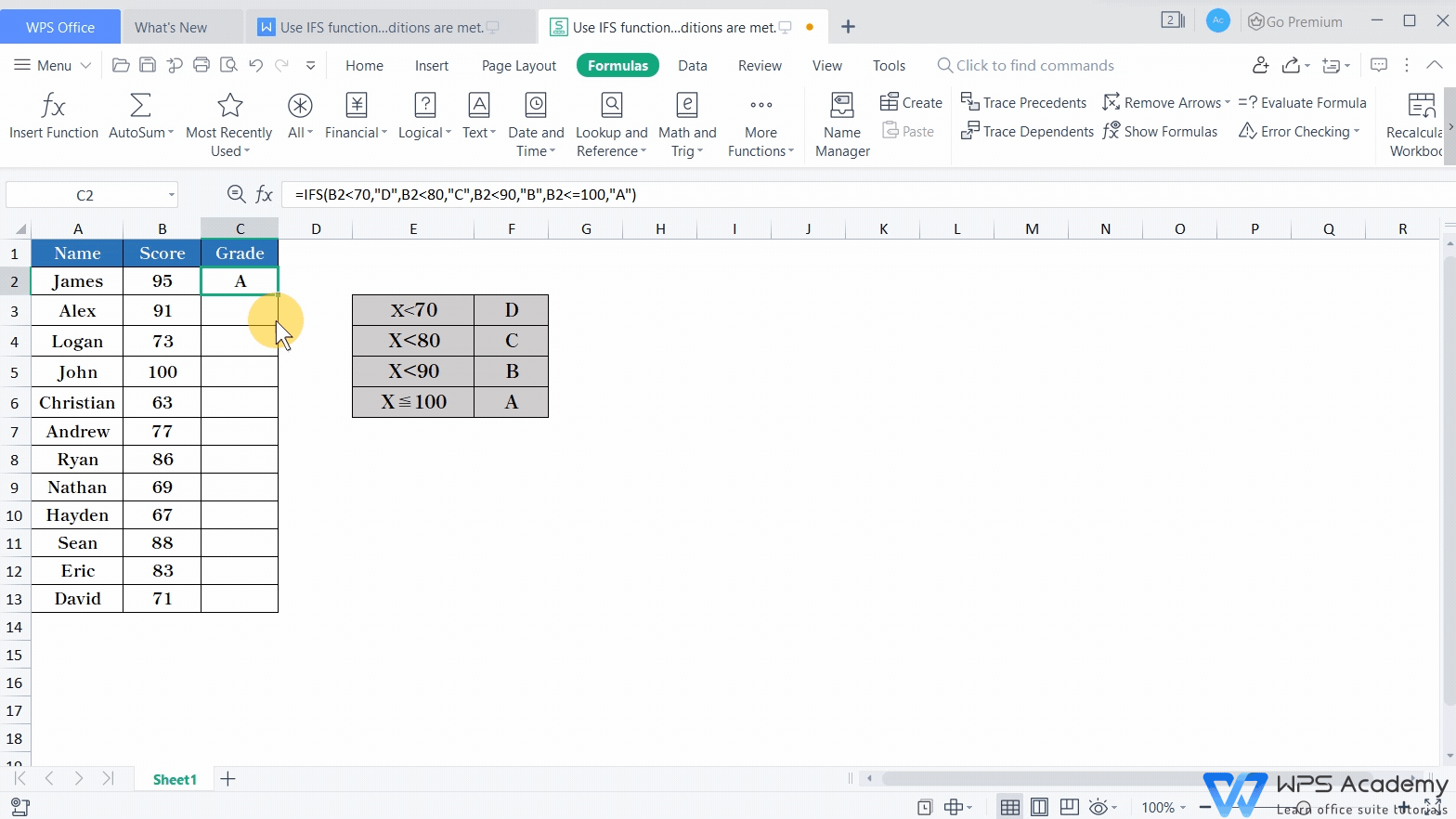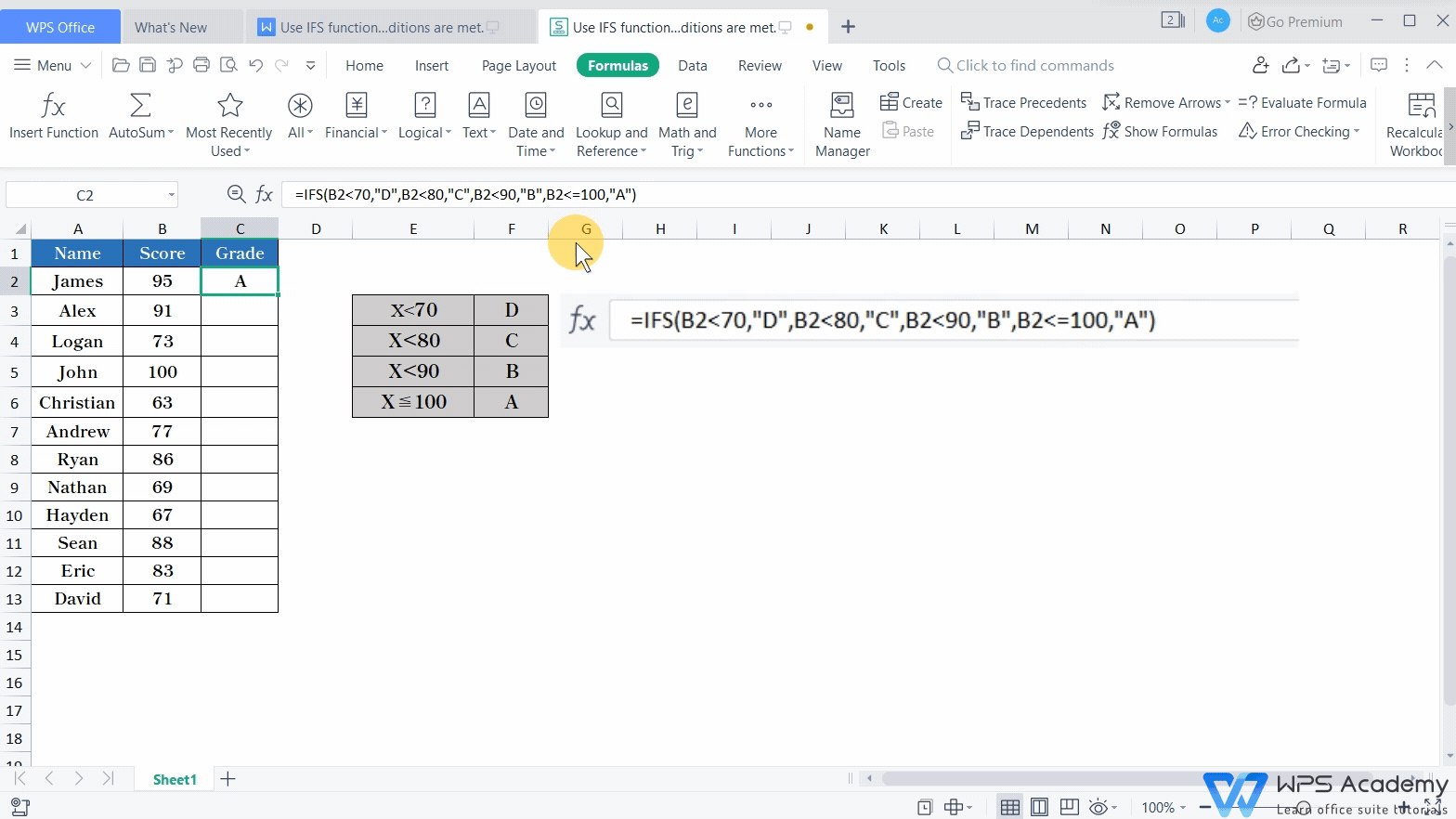# Use IFS function to check whether multiple conditions are met

Uploaded time: November 3, 2021 Difficulty: Intermediate# Use IFS function to check whether multiple conditions are met

When judging multiple conditions,  lengthy IF function will make it difficult for others to understand the logic when editing, here, IFS function can be put to good use.

The grammatical structure of IFS function is = IFS (logical _ test1, value _ if _ true1, [logical _ test2, value _ if _ true2], ...), making nesting layer by layer unnecessary, thus simplifying the formula.

Taking this form as an example, we would like to grade students' scores according to the four conditions of test scores on the right. First, select cell C2, click Insert Function under the Formulas tab and find the IFS function in the dialog box.

Every two parameters of the IFS function are set as a group, and the former Logical_test1 is the test condition. Here, we enter B2<70. The latter value_if_true1 means the result to be returned if logical_test1 evaluates to TRUE. Here we enter D. When the test result is FALSE, the IFS function will continue to judge the following test condition.We enter B2<80 in Logical_test2and enter C in value_if_true2.

Then, we enter “B2<90” in Logical_test3and enter B in value_if_true3.

Finally, enter “B2<=100” in Logical_test4and enter A in value_if_true4.

Then, click OK to complete the operation.The above formula first judges whether B2<70 is true, and if so, return to D

If B2<70 is not true, continue to judge whether B2<80 is true, and if so, return to C.

If B2<80 is not true, continue to judge whether B2<90 is true, and if so, return to B.

If B2<90 is not true, finally judge whether B2<=100 is true, if so, return to A.We can also specify TRUE in the last set of parameter test conditions to represent the situation that all the previous test conditions are not true.This is the basic use of IFS function. Did you get it